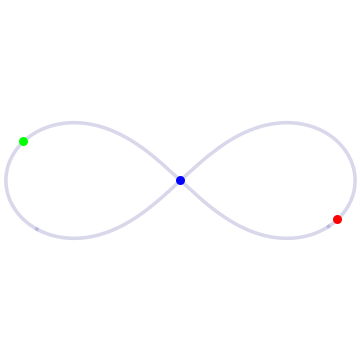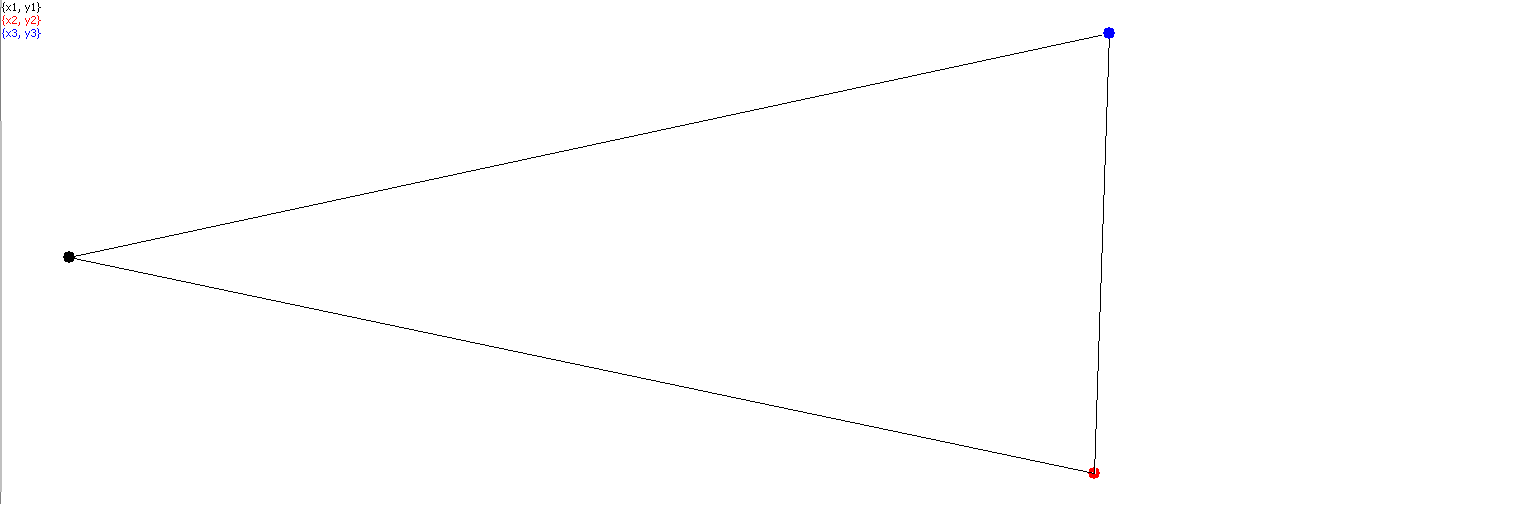Some animations, pictures, links and pointers to papers ... concerning the classical N-body problem and its solutions

 The figure eight solution:Three equal masses chase each other around a figure-eight shaped curve under the influence of their mutual gravitational attraction. Initial conditions (thanks to C Simo) : Positions: (x1,y1) = -(x2,y2); (x3,y3) = (0,0) ; (x1,y1) = (-0.97000436, 0.24308753) Velocites: (vx1,vy1) = (vx2, vy2) = -(vx3, vy3)/2; (vx3,vy3) = (0.93240737, 0.86473146) Parameters: m1 = m2 = m3 = 1 =G. ..viewed as a moving triangle:> Choreographies: Minton's build-your-own over 300 planar three-body choreographies, found by C. Simo; , implemented by P.Masson . (most have non-zero angular momentum) more ones mimicing platonic solids; C Moore's , etc The equal mass zero angular momentum three-body problem: Danya Rose's 2015 thesis and Bestiary More than 300 periodic solutions. Views from regularized shape space. Uses symplectic integration and regularized eqns 100s of periodic orbits by Suvakov & Dmitrasinovi. All start from the Euler config like the figure eight: one mass lying at the midpoint of the other two. Isosceles Solutions infinite families found by Nai-Chia Chen. (Hello Paul Klee!) Fujiwara, animations showing the three tangents theorem and other beautiful 3-body theorems Braking News non-collision periodic brake solutions by Li and Liao. More animation sites the 3-4-5 problem. Burtle-burtle's. Vanderbei's Open Problems , compiled by Albouy, Cabral, and Santos ***************** tours ; rather disorganized; some broken links; here primarily for giving public lectures ***************** Scholarpedia : 3-body problem -Chenciner N-body choreographies (-me) stuff posted before 2011and largely unedited since 5 Lectures on the N body Problem, Madrid ** fix link Richard !! ** (unpublished) An unpublished arxival version ``Figure 8s with three bodies'' (an earlier incomplete unpublished version of the eventual paper with Chenciner. See commentary below.) Commentary on the eight. Alain Chenciner and I rediscovered this surprising orbit for the Newtonian three body problem: with three equal point masses chase each other 'round a figure eight in the plane C. Simo has shown (numerically) that it is (KAM) stable: more precisely: as stable as a general periodic orbit in a 3 degree of freedom Hamiltonian system can be (pure imag. eigenvalues, torsion non-zero). Cris Moore , (Santa Fe inst.) had earlier found the same, via numerical integration and a gradient search. In a Physical Reviews Letters paper, 1993, vol 70. In 2003 Fujiwara and I gave an analytic proof that each of the eight's two lobes are convex. thanks to Carles Simo numerical wizard. (The initial positions are chosen to be collinear, with center of mass zero. The initial velocities are chosen so that the total linear and angular momenta are zero, and so that the total moment of inertia \$\Sigma m_a (x_a ^2 + y_a ^2)\$ is extremized. This leaves two free parameters represented by the velocity of mass 3.) A bit of history. The unpublished arxival version above predates the Annals paper with Chenciner by a few months. The `eight' of its title was in shape space not in inertial space. and was a different orbit from the eventual eight, an orbit whose existence is still in question. Chenciner and Venturelli discovered an error in the proof of `theorem 1' which claimed the existence of this orbit. Theorem 2 led to my paper with Chenciner. Andrea Venturelli, in his thesis under Chenciner, made use of some ideas here concerning using local perturbation analysis to delete collisions and thereby decreasing action, and concerning the constancy of energy along collision minimizers. A popular accounting of the eight and more new orbits can be found in the Notices article plus Casselman's commentary and pictures Choreographies, are orbits in which N planets chase each other around the same planar curve. The eight and Lagrange's orbit are choreographies. Soon after we rediscovered the eight solution, a horde of new solutions were discovered using the same ideas: variational methods plus symmetry. For the Newtonian potential case we lack existence proofs for all new solutions except the eight. In 2003 Ferrario and Terrracini proved the existence of some infinite families of choreographies. The breakthrough was Marchall's averaging of perturbations idea, as exposed by Chenciner's ICM notes All of these new variational minimizers EXCEPT the eight are unstable dynamically. **** overflow... non-collision 3-body brake (Nai-Chia Chen) 4 body collision families (Nai-Chia Chen) (both coded in 2014 by Nai-Chia Chen) isosceles families (Nai-Chia Chen; 2013). reminescent of Paul Klee